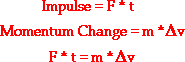# Mr. Malinen's Physics Class

## Welcome to Physics:

This website is provided as an additional resource to assist student learning and promote effective communication.  Please use this website to reference current and upcoming events, assignments, etc, as an aid to learning.

# Upcoming events and assignments:

Weekly assignments broken down by day/chapter/section/page/ and homework as appropriate

ex: 12/01/11 Momentum Ch. 3 Virtual Lab 6 problems 1-10 pg. 35

# Physics in the Real World

### Real-World Applications

In a collision, an object experiences a force for a given amount of time that results in its mass undergoing a change in velocity (i.e., that results in a momentum change).

There are four physical quantities mentioned in the above statement - force, time, mass, and velocity change. The force multiplied by the time is known as the impulse and the mass multiplied by the velocity change is known as the change in momentum. The impulse experienced by an object is always equal to the change in its momentum. In terms of equations, this was expressed asThis is known as the impulse-momentum change theorem.In this lesson, we will examine some real-world applications of the impulse-momentum change theorem. We will examine some physics in action in the real world. In particular, we will focus upon

• the effect of collision time upon the amount of force an object experiences, and
• the effect of rebounding upon the velocity change and hence the amount of force an object experiences.

As an effort is made to apply the impulse-momentum change theorem to a variety of real-world situations, keep in mind that the goal is to use the equation as a guide to thinking about how an alteration in the value of one variable might effect the value of another variable.